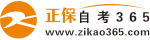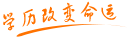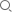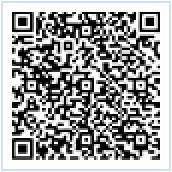#24小时客服：4008135555/010-82335555# 自考“C语言程序设计”模拟试题六答案

2007年05月29日    来源：   字体：   打印

一、单项选择题

1. C    2. C   3. C   4. C D   5. C   6. B   7. D   8. C

9. B    10. C  11. B  12.A   13  D   14. B  15. A   16.   A.

17.  C   18. B   19. A.  20. C.

二、写出下列程序的运行结果

1.10  9

2.S=9

3. 1，5

4.19

5.40

三、判断题

1.  对   2.  不对   3.  不对   4.  对    5.不对

6.  对   7.  不对   8.  不对   9.  不对  10.不对

四、编程题

1.  main（）

{   int  m，n；

int  a，b=0；

for（a=0；a<20；a++）

{   scanf（“%d”，&m[a]）；

if（a%2= =1）

{   n[b]=m[a]；

b++；      }

}

}

2. main（）

{   int  m={ 3，-30，20，6，77，2，0，-4，-7，99}，max，min；

max=min=m；

for（a=0；a<10；a++）

{   if（m[a]>max）

max=m[a]；

if（m[a]<min）

min=m[a]；

}

printf（“%d，%d”，max，min）；

}

3. int  stu（int a，int b）

{   int c；

c=a*a+b*b；

return c；     }

main（）

{   int  x，y，z；

scanf（“%d，%d”，&x，&y）；

z=stu（x，y）；

printf（“%d”，z）；    }

4.main（）

{   FILE  *fp；

Char ch；

fp=fopen（“write.txt”，“r”）；

ch=fgetc （fp）；

while（ch！= EOF）

{   putchar（ch）；

ch=fgetc（fp）；   }

fclose（fp）；

}

5.main（）

{

int  sum1=0，sum2=0，a，b；

for（a=1；a<=100；a++）

if（a%2= =0）  if（a%2= =0）

sum1+=a；

else

sum2+=a；

printf（“%d，%d”，sum1，sum2）；

}

• 热门课程
• 报名咨询• 购物车
• APP下载扫一扫，立即下载

安卓版本：

苹果版本：

开发者：北京正保自考教育科技有限公司

应用涉及权限：查看权限>>

APP隐私策略：查看政策>>

• 公众号扫一扫，立即关注

• 微信群进群领试题/资料